Latest SSC jobs   »   Reasoning Quiz for SSC CGL Exam...

# Reasoning Quiz for SSC CGL Exam 2020: 15th February 2020 For Analogy, Counting Figures

Q1. Rs 1,875 is divided among A, B and C in such a way that A’s share is half of the combined share of B and C, and B’s share is one-fourth of the combined share of A and C. By what amount is C’s share more than that of A ?
(a) Rs 500
(b) Rs 225
(c) Rs 250
(d) Rs 200

Q2. Select the word-pair in which the two words are related in the same way as are the two words in the following word pair
Book : Thesaurus
(a) Tree : forest
(b) Reptile : Python
(c) Furniture : Wood
(d) Tennis : Ball

Q3. Which two signs should be interchanged in the following equation to make it correct?
12 – 8 + 12 × 9 ÷ 3 = 9
(a) + and ÷
(b) + and ×
(c) – and ÷
(d) + and –

Q4. How many squares are there in the following figure?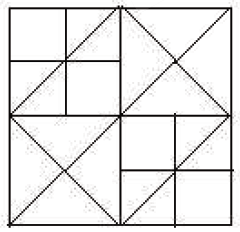(a) 12
(b) 16
(c) 13
(d) 14Q5. Select the correct mirror image of the given figure when the mirror is placed to the right of the figure.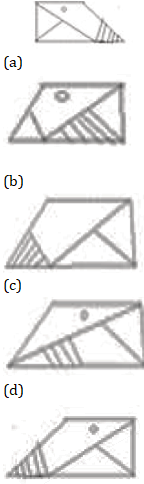Q6. Which two signs should be interchanged in the following equation to make it correct?
18 + 6 – 6 ÷ 3 × 3 = 6
(a) + and –
(b) + and ÷
(c) – and ÷
(d) + and ×

Q7. Arrange the following words in a logical and meaningful order
1. medicine
2. Diagnosis
3. Prescription
4. Illness
5. Recovery
6. Doctor
(a) 4, 6, 2, 3, 1, 5
(b) 4, 6, 1, 3, 2, 5
(c) 2, 6, 4, 1, 3, 5
(d) 4, 6, 3, 2, 1, 5

Q8. Select the figure that will come next in the following figure series ;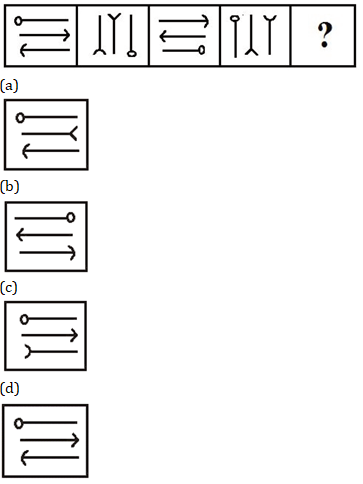Q9. Three of the following four numbers are alike in a certain way and one is different. Pick the number that is different from the rest.
(a) 12
(b) 14
(c) 56
(d) 30

Q10. If BACK is coded as 11312 and CAKE is coded as 51113, then how will MADE be coded as ?
(a) 51413
(b) 54113
(c) 31145
(d) 13145

#### Solutions: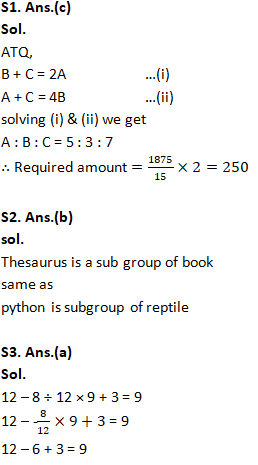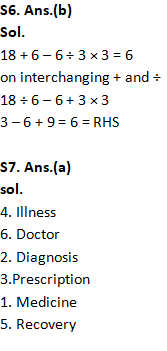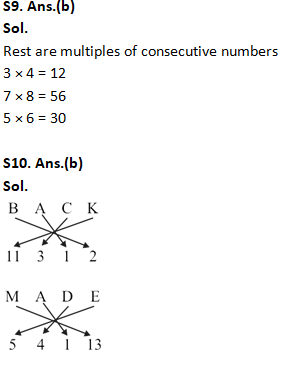You may also like to read:

#### Congratulations!General Awareness & Science Capsule PDF